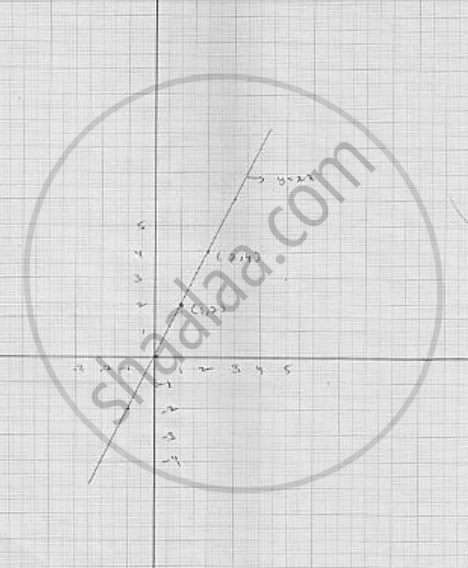# Draw the Graph of the Following Linear Equation in Two Variable : Y = 2x - Mathematics

Draw the graph of  the following linear equation in two variable : y = 2x

#### Solution

We have
y = 2 x                       ...................... ( 1)
Putting  x  = 0  ,we get  y = 2  × 0   = 0
Putting  x = 1  we get y = 2 × 1 = 2
Thus, we get the following table giving the two points on the line represented by the equation  y = 2x     Graph for the equation  y = 2xConcept: Graph of a Linear Equation in Two Variables
Is there an error in this question or solution?

#### APPEARS IN

RD Sharma Mathematics for Class 9
Chapter 7 Linear Equations in Two Variables
Exercise 7.3 | Q 1.4

Share